# Algebra - Rationalize The Denominator

in STEMGeeks18 days ago

Hi everyone. This mathematics post is focused on rationalizing the denominator. It is an algebra technique that is used to remove square roots from the denominator of a fraction.

Math text rendered with QuickLatex.com.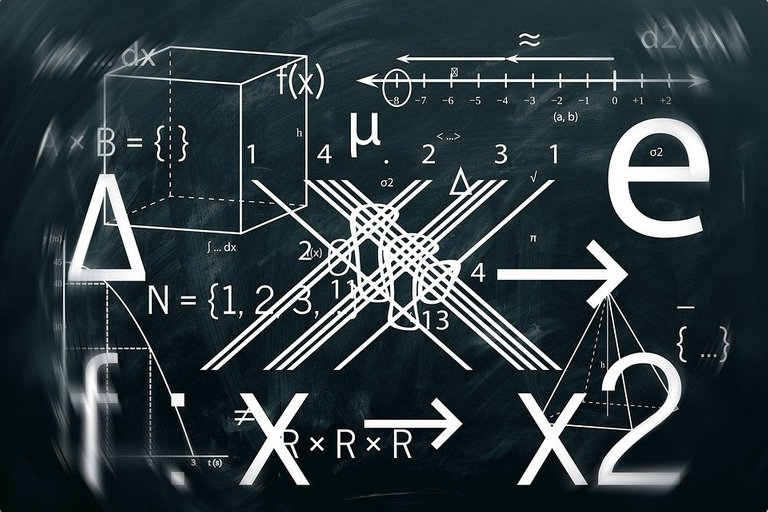Pixabay Image Source

## Short Review Of Exponent Laws and Square Roots

Given two numbers with a common base and different exponents being multiplied together, they can be combined into a single base where the different exponents are added together.

As an example if you have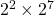, this can be rewritten into a single base and a single exponent as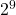. The exponents of 2 and 7 are added together to obtain the sum of 9.

In general, you have: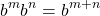where `b` is the number base, `m` is the first number exponent and `n` is the second number exponent.

Square Root As The Exponent Of Half

A concept that some math students forget or are not aware of is that the square root as an exponent is one half.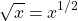The square root of a product such as `xy` is the same as the square root of `x` multiplied by the square root of `y`. This is based on the exponent laws.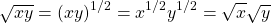In summary for this section, the result worth remembering is: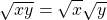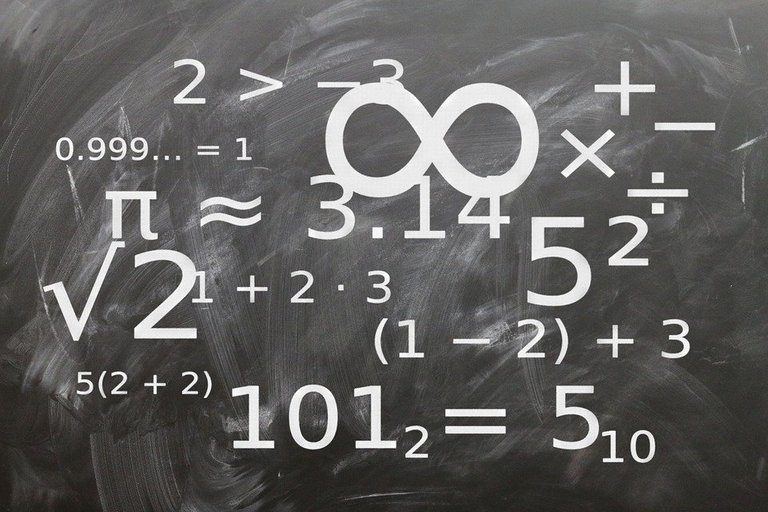Pixabay Image Source

## No Square Roots On The Bottom

When it comes to mathematics formatting there are some unspoken mathematics style rules. It is common to prefer no square root values in the denominator of fractions.

We are not there in rationalizing the denominator and removing square roots yet. This simple of trick of "multiplying by one" to the fraction is a nice way of removing square root values from the bottom of fractions.

Example One

Sometimes you may obtain answers such as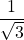. The square root of 3 is on the denominator. To make this fraction into more a "valid" answer we multiplying by "one" by multiplying top and bottom by the square root of 3.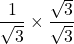The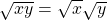result from earlier is applied to the bottom of the fraction.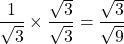The square root of 9 is 3. Another way is to use exponents instead of square roots on the denominator.

Note that: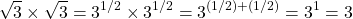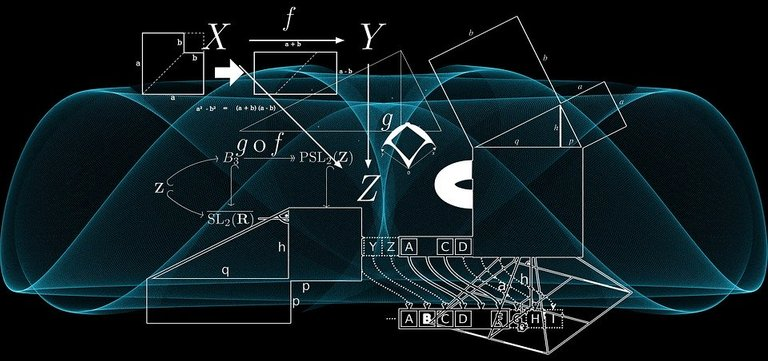Pixabay Image Source

## The Use Of Conjugates In Rationalizing The Denominator

In the earlier section there was only on term in the square root. What if there two terms (binomial) in a square root? How can we remove square roots of binomials?

We do the multiply by one trick along with the use of conjugates.

The Conjugate Of A Binomial

If you have a binomial of the form `a + bz`, the conjugate would be `a - bz`. The conjugate of `a - bz` would be `a - (-bz)` or `a + bz`.

As one example, the conjugate of 5 + 7x would be 5 - 7x.

The conjugate of 3 - x is 3 + x.

Rationalizing The Denominator With Conjugates

This rationalizing the denominator algebra technique will be shown through an example.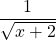To remove the square root below, multiply by "one" with the conjugate of x plus the square root of 2 on the numerator and denominator.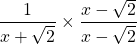The numerator of this fraction is just x minus the square root of 2. The bottom is a product of two binomials. In fact, this product of binomials is the factored version of the difference of squares factoring technique.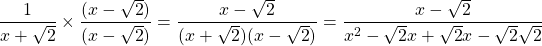In the bottom of the part of the denominator the middle two terms out of the four cancel each other out. The last term of two square root of two behind the negative becomes 2 (with square root exponent laws).

In summary you have: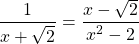Another Example

This next example features two square roots in a binomial. What if we wanted to remove the two square roots on the bottom of the fraction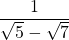To rationalize the denominator, we still use conjugates. The conjugate of the square root of 5 minus the square root of 7 is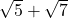. This conjugate is used when multiplying the fraction by "one".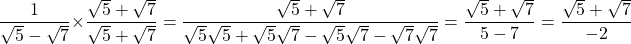Pixabay Image Source